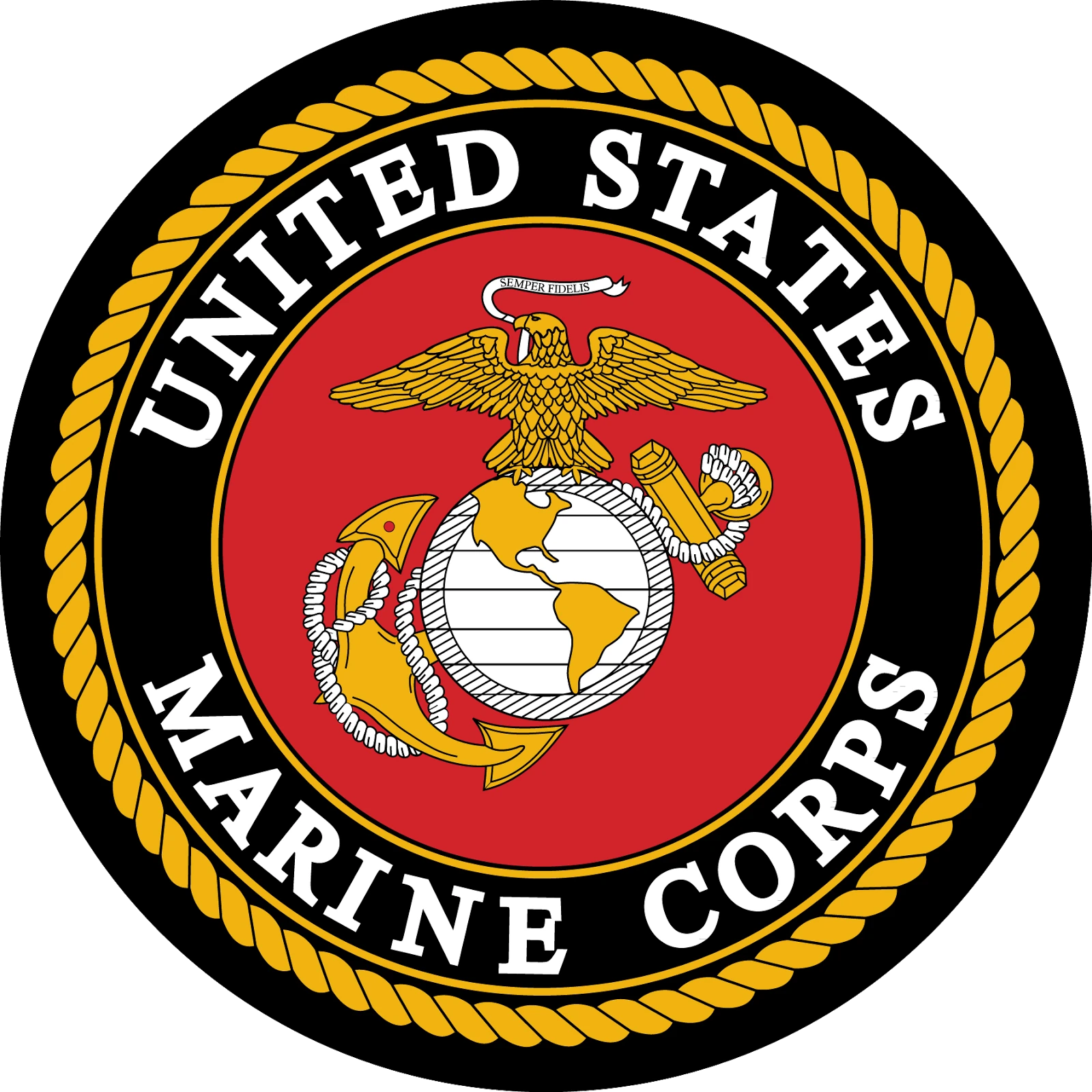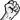{{#infoboxbuilder: | 0:Theme = default | 0:CustomModule = | 0:MainImageCaption = On | 0:ToggleContentLongerThan = 1000

| 1:Type = Footer

```| 1:Value =
```

| 2:Type = Title

```| 2:Value = USMC
```

| 3:Type = MainImage

```| 3:Value =| 3:Label = The USMC' insignia.
```

| 4:Type = Line

```| 4:Label = Games
| 4:Value =IMIC
```

| 5:Type = Line

```| 5:Label = Type
| 5:Value =  Regular Army
```

| 6:Type = Line

```| 6:Label = Affiliation
| 6:Value =  Unknown
```

| 7:Type = Line

```| 7:Label =
| 7:Value =
```

| 8:Type = Line

```| 8:Label =
| 8:Value =
```

| 9:Type = Line

```| 9:Label =
| 9:Value =
```

| 10:Type = Line

```| 10:Label =
| 10:Value =
```

| 11:Type = Line

```| 11:Label =
| 11:Value =
```

| 12:Type = Line

```| 12:Label =
| 12:Value =
```

| 13:Type = Line

```| 13:Label =
| 13:Value =
```

| 14:Type = Line

```| 14:Label =
| 14:Value =
```

| 15:Type = Line

```| 15:Label =
| 15:Value =
```

| 16:Type = Line

```| 16:Label =
| 16:Value =
```

| 17:Type = Line

```| 17:Label =
| 17:Value =
```

| 18:Type = Line

```| 18:Label =
| 18:Value =
```

| 19:Type = Image

```| 19:Value =
```

| 20:Type = Footer

```| 20:Value =
```

}} The United States Marine Corps (USMC) is a branch of the United States Armed Forces. They are the opponents of the Insurgent Forces in Insurgency: Modern Infantry Combat.